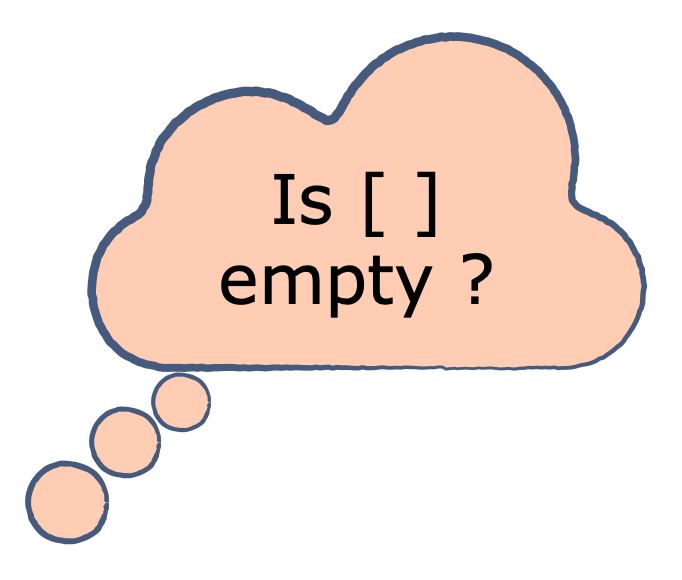Related Tags

python
list
operators

# How to check if a Python list is emptyEducative Answers Team

A list is a frequently used data structure in Python. Therefore, users often need to determine if a list is empty or not.

There are several ways to do this. Some of the ways may appear to be more explicit while others take relatively less time to execute and hence, are endorsed by the developers of Python.## Possible techniques

### 1. Using the not operator

First, let’s take a look at the most “Pythonic” and recommended way to do determine if a list is empty. An empty data structure is always evaluated to false in Python, ​and the following method relies on this boolean interpretation of a data structure in the language.

As mentioned earlier, an empty list evaluates to false; when the not operator is applied on false, the result is true and hence, the code inside the if condition is executed.​

Pro

• Computationally faster than all the other ways.
Con

• It may seem like the list is a boolean.
# RECOMMENDED
empty_list = []
if not empty_list:
print('The list is empty!')
else:
print('The list contains something.')

### 2. Using the len function

Now, let’s look at a method that seems obvious: the built-in length function​.

len returns 0 if the list is empty.

Pro

• Easy to understand.
Con

• Computationally slower. First, the length function is computed and then the comparison is made.
empty_list = []
if len(empty_list) == 0:
print('The list is empty!')
else:
print('The list contains something.')


### 3. Comparison with an ​empty list

Lastly, consider another approach that seems pretty straightforward.

Here, compare_with is another empty list and we compare our own list with it.

Pro

• Easy to understand.
Con

• Computationally slower. Here an empty list is created and then a comparison is done.
empty_list = []
compare_with = []
if empty_list == compare_with:
print('The list is empty!')
else:
print('The list contains something.')

RELATED TAGS

python
list
operators